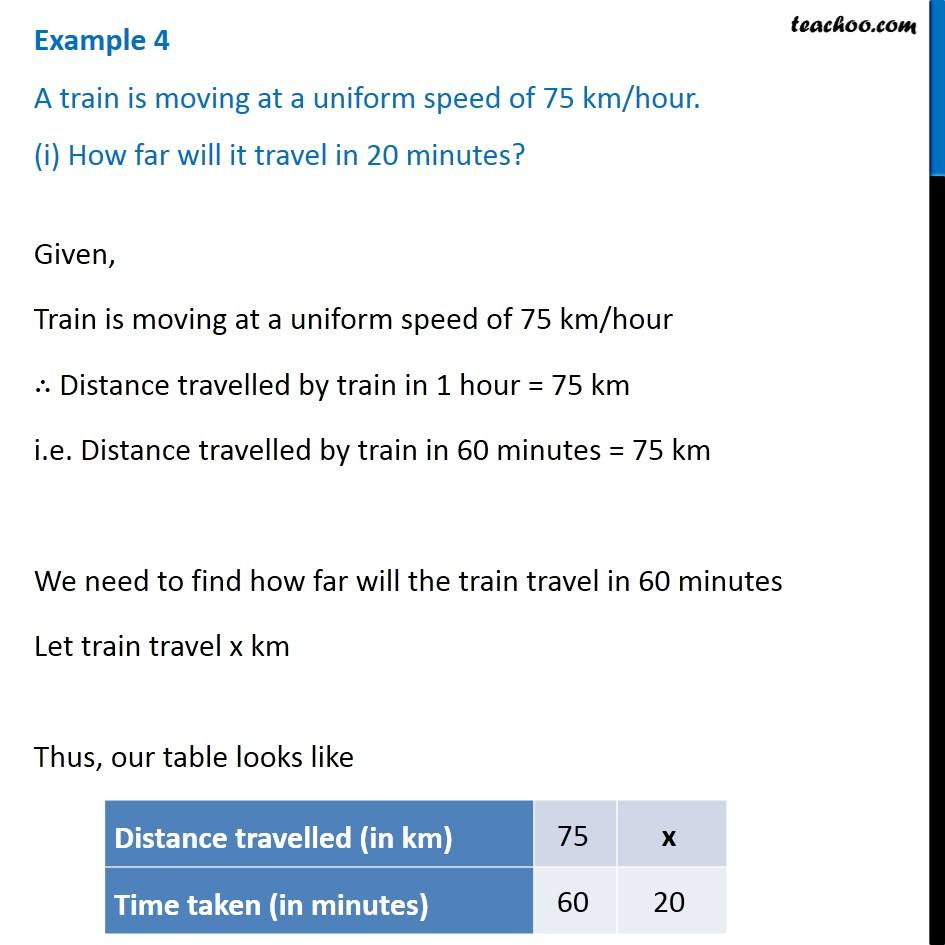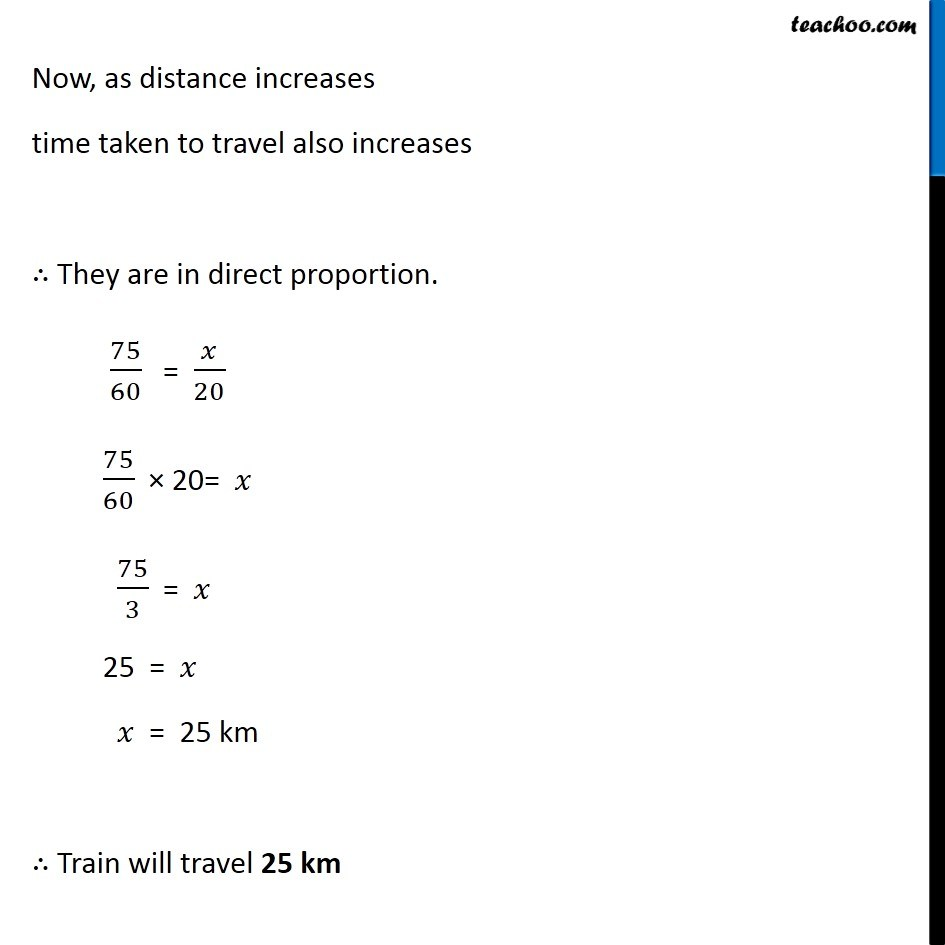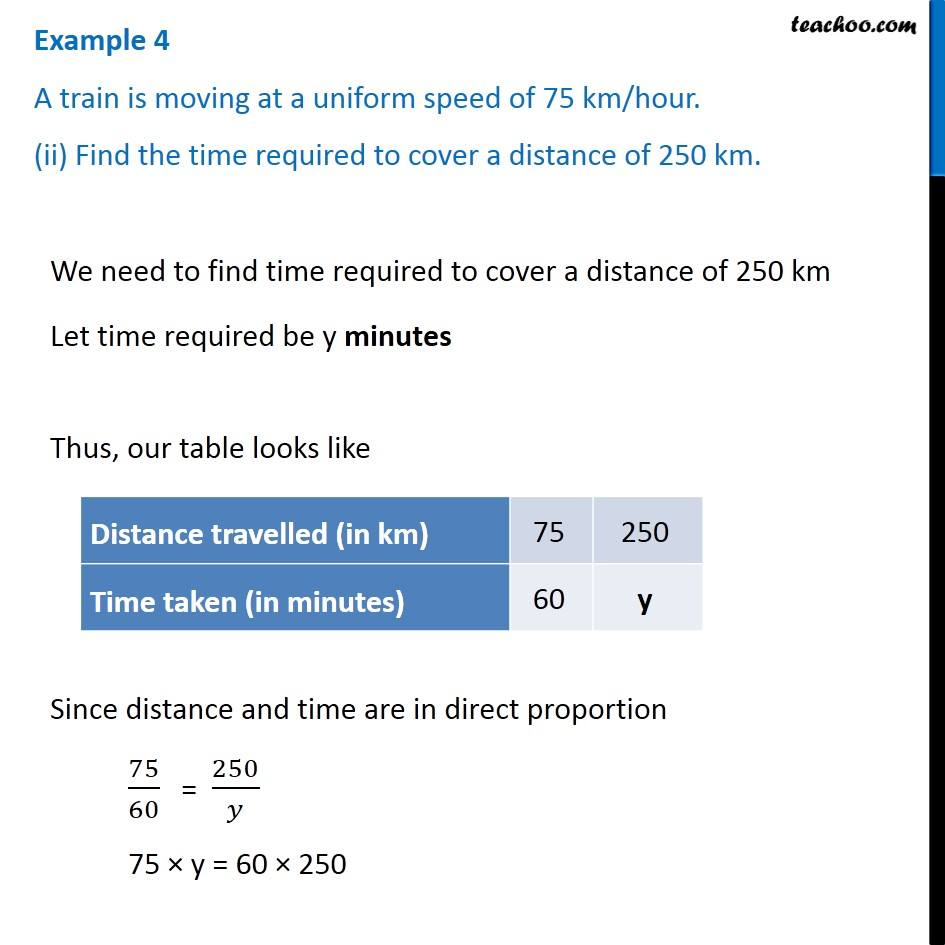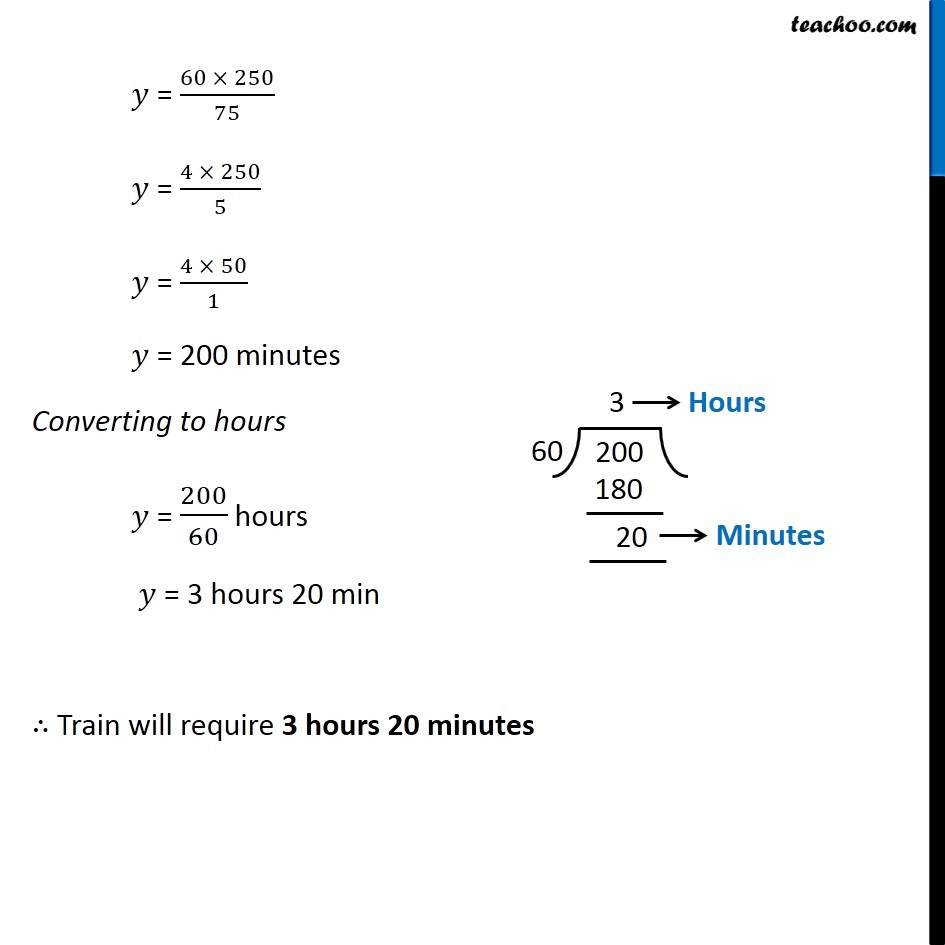Subscribe to our Youtube Channel - https://you.tube/teachoo

1. Chapter 13 Class 8 Direct and Inverse Proportions
2. Concept wise
3. Direct Proportion

Transcript

Example 4 A train is moving at a uniform speed of 75 km/hour. (i) How far will it travel in 20 minutes? Given, Train is moving at a uniform speed of 75 km/hour ∴ Distance travelled by train in 1 hour = 75 km i.e. Distance travelled by train in 60 minutes = 75 km We need to find how far will the train travel in 60 minutes Let train travel x km Thus, our table looks like Now, as distance increases time taken to travel also increases ∴ They are in direct proportion. 75/60 = 𝑥/20 75/60 × 20= 𝑥 75/3 = 𝑥 25 = 𝑥 𝑥 = 25 km ∴ Train will travel 25 km Example 4 A train is moving at a uniform speed of 75 km/hour. (ii) Find the time required to cover a distance of 250 km. We need to find time required to cover a distance of 250 km Let time required be y minutes Thus, our table looks like Since distance and time are in direct proportion 75/60 = 250/𝑦 75 × y = 60 × 250 𝑦 = (60 × 250)/75 𝑦 = (4 × 250)/5 𝑦 = (4 × 50)/1 𝑦 = 200 minutes Converting to hours 𝑦 = 200/60 hours 𝑦 = 3 hours 20 min ∴ Train will require 3 hours 20 minutes

Direct Proportion